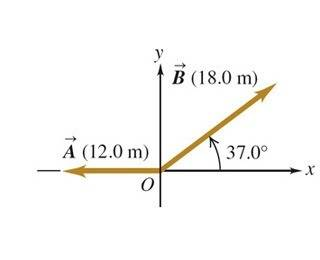# Subtracting this vector - as opposed to adding it

## Homework Statement

Sketch the vector difference D = A - B

I have found F = A + B.

## Homework Equations

A(x) = AcosTHETA
A(y) = AsinTHETA
B(x) = BcosTHETA
A(y) = BsinTHETA

R = sqrt(rx^2 + ry^2)

## The Attempt at a Solution

As opposed to the F = A + B I have tried changing the signs of B or multiplying B(x) = -BcosTHETA etcetera and I can't come with the right solution. Do I need to put the angle 37 degrees in the second quadrant? I am confused as how to find -B as A is going to be the same as the first equation (F = A + B).

#### Attachments

•Figure-01.37.jpg
7.3 KB · Views: 391

SammyS
Staff Emeritus
Homework Helper
Gold Member

## Homework Statement

Sketch the vector difference D = A - B

I have found F = A + B.

## Homework Equations

A(x) = AcosTHETA
A(y) = AsinTHETA
B(x) = BcosTHETA
B(y) = BsinTHETA

R = sqrt(rx^2 + ry^2)

## The Attempt at a Solution

As opposed to the F = A + B I have tried changing the signs of B or multiplying B(x) = -BcosTHETA etcetera and I can't come with the right solution. Do I need to put the angle 37 degrees in the second quadrant? I am confused as how to find -B as A is going to be the same as the first equation (F = A + B).Hello rpgnick85. Welcome to PF !

You would have
-Bx = -Bcosθ

-By = -Bsinθ​
That puts -B in the third Quadrant, doesn't it?

-Bx = -Bcosθ

-By = -Bsinθ

Does that mean that I would do -18cos(217) for my first value?

SammyS
Staff Emeritus
Homework Helper
Gold Member
-Bx = -Bcosθ

-By = -Bsinθ

Does that mean that I would do -18cos(217) for my first value?
No.

The x component of -B is either 18cos(217°) or -18cos(37°) .

alright so when i use -18cos37 and -18sin37 for my Bx and By values respectively and 12cos180 and 12sin180 for my Ax an Ay values I am coming up with R= 28.5126 and θ=22.33° which is incorrect